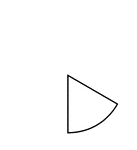### One Sixth of a Fraction Pie

One sixth of a circle.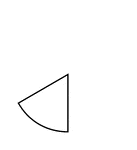### One Sixth of a Fraction Pie

One sixth of a circle.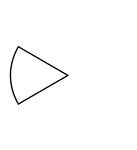### One Sixth of a Fraction Pie

One sixth of a circle.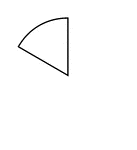### One Sixth of a Fraction Pie

One sixth of a circle.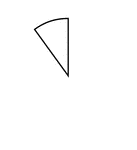### One Tenhs of a Fraction Pie

One tenth of a circle.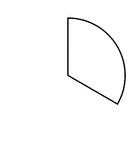### One Third of a Fraction Pie

One third of a circle.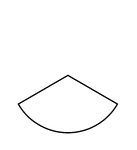### One Third of a Fraction Pie

One third of a circle.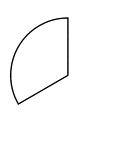### One Third of a Fraction Pie

One third of a circle.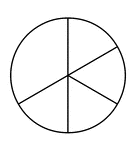### One Third and Four Sixths of a Pie Fraction

A circle subdivided into one third and four sixths.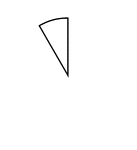### One Twelfth of a Fraction Pie

One twelfth of a circle.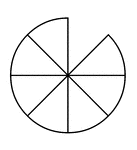### Seven Eighths of a Fraction Pie

Seven eighths of a circle.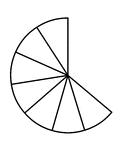### Seven Elevenths of a Fraction Pie

Seven elevenths of a circle.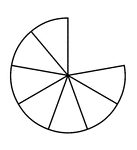### Seven Ninths of a Fraction Pie

Seven ninths of a circle.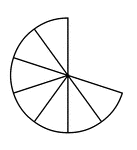### Seven Tenths of a Fraction Pie

Seven tenths of a circle.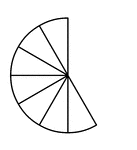### Seven Twelfths of a Fraction Pie

Seven twelfths of a circle.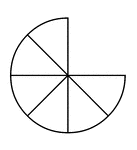### Six Eighths of a Fraction Pie

Six eighths of a circle.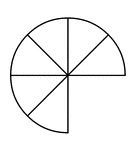### Six Eighths of a Fraction Pie

Six eighths of a circle.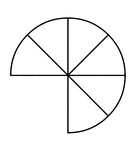### Six Eighths of a Fraction Pie

Six eighths of a circle.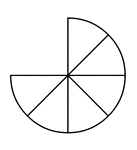### Six Eighths of a Fraction Pie

Six eighths of a circle.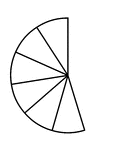### Six Elevenths of a Fraction Pie

Six elevenths of a circle.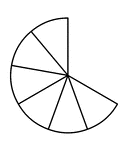### Six Ninths of a Fraction Pie

Six ninths of a circle.### Six Sevenths of a Fraction Pie

Six sevenths of a circle.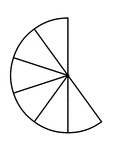### Six Tenths of a Fraction Pie

Six tenths of a circle.### Six Twelfths of a Fraction Pie

Six twelfths of a circle.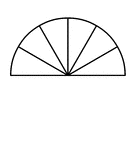### Six Twelfths of a Fraction Pie

Six twelfths of a circle.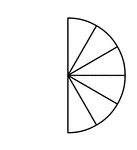### Six Twelfths of a Fraction Pie

Six twelfths of a circle.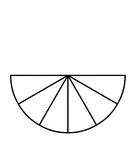### Six Twelfths of a Fraction Pie

Six twelfths of a circle.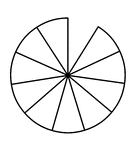### Ten Elevenths of a Fraction Pie

Ten elevenths of a circle.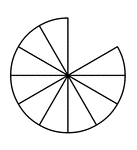### Ten Twelfths of a Fraction Pie

Ten twelfths of a circle.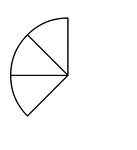### Three Eighths of a Fraction Pie

Three eighths of a circle.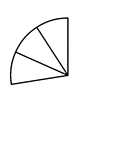### Three Elevenths of a Fraction Pie

Three elevenths of a circle.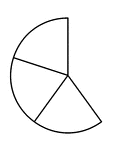### Three Fifths of a Fraction Pie

Three fifths of a circle.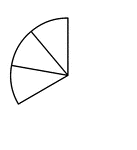### Three Ninths of a Fraction Pie

Three ninths of a circle.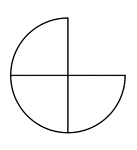### Three Quarters of a Fraction Pie

Three quarters of a circle.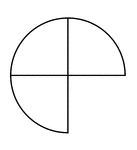### Three Quarters of a Fraction Pie

Three quarters of a circle.### Three Quarters of a Fraction Pie

Three quarters of a circle.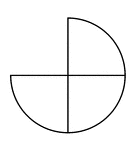### Three Quarters of a Fraction Pie

Three quarters of a circle.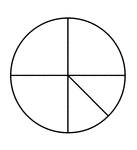### Three Quarters and Two Eighths of a Pie Fraction

A circle subdivided into three quarters and two eighths.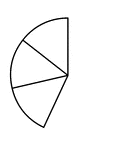### Three Sevenths of a Fraction Pie

Three sevenths of a circle.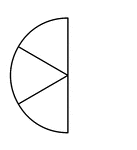### Three Sixths of a Fraction Pie

Three sixths of a circle.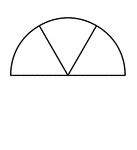### Three Sixths of a Fraction Pie

Three sixths of a circle.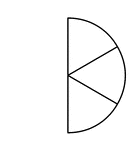### Three Sixths of a Fraction Pie

Three sixths of a circle.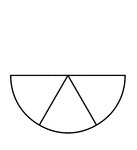### Three Sixths of a Fraction Pie

Three sixths of a circle.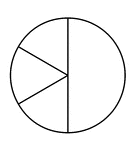### Three Sixths and One Half of a Pie Fraction

A circle subdivided into three sixths and one half.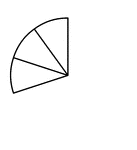### Three Tenths of a Fraction Pie

Three tenths of a circle.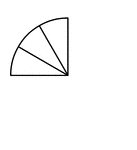### Three Twelfths of a Fraction Pie

Three twelfths of a circle.### Three Twelfths of a Fraction Pie

Three twelfths of a circle.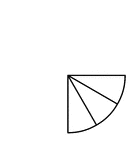### Three Twelfths of a Fraction Pie

Three twelfths of a circle.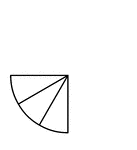### Three Twelfths of a Fraction Pie

Three twelfths of a circle.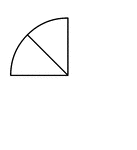### Two Eighths of a Fraction Pie

Two eighths of a circle.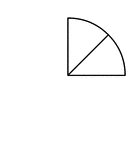### Two Eighths of a Fraction Pie

Two eighths of a circle.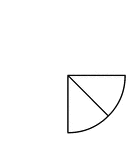### Two Eighths of a Fraction Pie

Two eighths of a circle.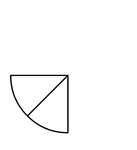### Two Eighths of a Fraction Pie

Two eighths of a circle.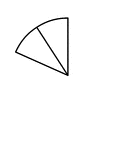### Two Elevenths of a Fraction Pie

Two elevenths of a circle.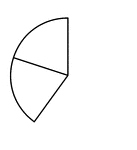### Two Fifths of a Fraction Pie

Two fifths of a circle.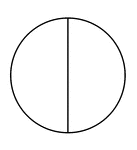### Two Halves of a Fraction Pie

A circle divided in half vertically.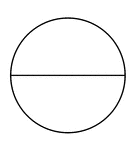### Two Halves of a Fraction Pie

A circle divided in half horizontally.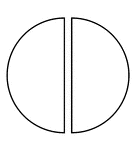### Two Halves of a Fraction Pie

A circle divided in half vertically separated.### Two Halves of a Fraction Pie

A circle divided in half horizontally separated.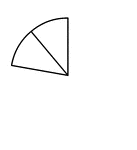### Two Ninths of a Fraction Pie

Two ninths of a circle.